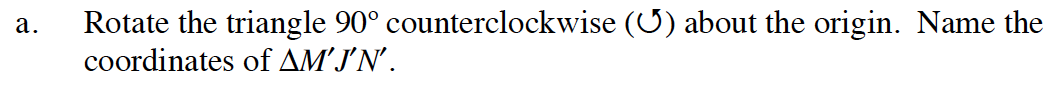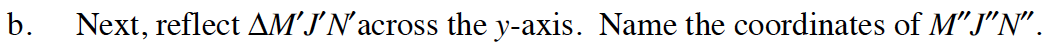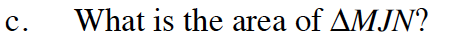Home > GB8I > Chapter cc46 > Lesson cc46.4.2 > Problem6-139

6-139.
1. Plot ΔMJN on graph paper with points M(3, 3), J(1, 1), and N(6, 1). 6-139 HW eTool (Desmos). Homework Help ✎

1. Rotate the triangle 90º counterclockwise (↺) about the origin. Name the coordinates of ΔMJN′.

2. Next, reflect ΔMJN′ across the y-axis. Name the coordinates of MJN″.

3. What is the area of ΔMJN?Use the eTool to complete the transformation. Notice how the points have changed. Pay attention closely to point N.
Notice that the y- coordinate has been multiplied by −1 and the x- and y-coordinates are interchanged.Use the eTool. Notice how the coordinates compare to the coordinates of ΔM''J''N''. What happens to the points when you translate across the y-axis?

M''(3, 3), J''(1, 1), N''(1, 6)What is the length of the base, JN? What is the height from point M to base JN? Use the area formula for triangles.

Use the eTool below to solve the problem.
Click the link at right for the full version of the eTool: INT1 6-139 HW eTool